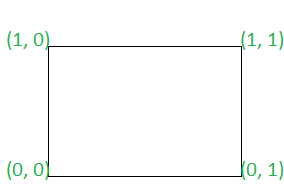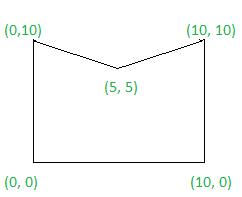# Check if given polygon is a convex polygon or not

• Difficulty Level : Medium
• Last Updated : 09 Aug, 2021

Given a 2D array point[][] with each row of the form {X, Y}, representing the co-ordinates of a polygon in either clockwise or counterclockwise sequence, the task is to check if the polygon is a convex polygon or not. If found to be true, then print “Yes” . Otherwise, print “No”.

In a convex polygon, all interior angles are less than or equal to 180 degrees

Examples:

Input: arr[] = { (0, 0), (0, 1), (1, 1), (1, 0) }
Output: Yes
Explanation:Since all interior angles of the polygon are less than 180 degrees. Therefore, the required output is Yes.

Input : arr[] = {(0, 0), (0, 10), (5, 5), (10, 10), (10, 0)}
Output : No
Explanation:Since all interior angles of the polygon are not less than 180 degrees. Therefore, the required output is No.

Approach: Follow the steps below to solve the problem:

• Traverse the array and check if direction of cross product of any two adjacent sides of the polygon are same or not. If found to be true, then print “Yes”.
• Otherwise, print “No”

Below is the implementation of the above approach:

## C++

 `// C++ program to implement``// the above approach` `#include ``using` `namespace` `std;` `// Utility function to find cross product``// of two vectors``int` `CrossProduct(vector >& A)``{``    ``// Stores coefficient of X``    ``// direction of vector AA``    ``int` `X1 = (A - A);` `    ``// Stores coefficient of Y``    ``// direction of vector AA``    ``int` `Y1 = (A - A);` `    ``// Stores coefficient of X``    ``// direction of vector AA``    ``int` `X2 = (A - A);` `    ``// Stores coefficient of Y``    ``// direction of vector AA``    ``int` `Y2 = (A - A);` `    ``// Return cross product``    ``return` `(X1 * Y2 - Y1 * X2);``}` `// Function to check if the polygon is``// convex polygon or not``bool` `isConvex(vector >& points)``{``    ``// Stores count of``    ``// edges in polygon``    ``int` `N = points.size();` `    ``// Stores direction of cross product``    ``// of previous traversed edges``    ``int` `prev = 0;` `    ``// Stores direction of cross product``    ``// of current traversed edges``    ``int` `curr = 0;` `    ``// Traverse the array``    ``for` `(``int` `i = 0; i < N; i++) {` `        ``// Stores three adjacent edges``        ``// of the polygon``        ``vector > temp``            ``= { points[i],``                ``points[(i + 1) % N],``                ``points[(i + 2) % N] };` `        ``// Update curr``        ``curr = CrossProduct(temp);` `        ``// If curr is not equal to 0``        ``if` `(curr != 0) {` `            ``// If direction of cross product of``            ``// all adjacent edges are not same``            ``if` `(curr * prev < 0) {``                ``return` `false``;``            ``}``            ``else` `{``                ``// Update curr``                ``prev = curr;``            ``}``        ``}``    ``}``    ``return` `true``;``}` `// Driver code``int` `main()``{``    ``vector > points``        ``= { { 0, 0 }, { 0, 1 },``            ``{ 1, 1 }, { 1, 0 } };` `    ``if` `(isConvex(points)) {``        ``cout << ``"Yes"``             ``<< ``"\n"``;``    ``}``    ``else` `{``        ``cout << ``"No"``             ``<< ``"\n"``;``    ``}``}`

## Java

 `// Java program to implement``// the above approach``class` `GFG``{``  ` `// Utility function to find cross product``// of two vectors``static` `int` `CrossProduct(``int` `A[][])``{``    ``// Stores coefficient of X``    ``// direction of vector AA``    ``int` `X1 = (A[``1``][``0``] - A[``0``][``0``]);` `    ``// Stores coefficient of Y``    ``// direction of vector AA``    ``int` `Y1 = (A[``1``][``1``] - A[``0``][``1``]);` `    ``// Stores coefficient of X``    ``// direction of vector AA``    ``int` `X2 = (A[``2``][``0``] - A[``0``][``0``]);` `    ``// Stores coefficient of Y``    ``// direction of vector AA``    ``int` `Y2 = (A[``2``][``1``] - A[``0``][``1``]);` `    ``// Return cross product``    ``return` `(X1 * Y2 - Y1 * X2);``}` `// Function to check if the polygon is``// convex polygon or not``static` `boolean` `isConvex(``int` `points[][])``{``    ``// Stores count of``    ``// edges in polygon``    ``int` `N = points.length;` `    ``// Stores direction of cross product``    ``// of previous traversed edges``    ``int` `prev = ``0``;` `    ``// Stores direction of cross product``    ``// of current traversed edges``    ``int` `curr = ``0``;` `    ``// Traverse the array``    ``for` `(``int` `i = ``0``; i < N; i++) {` `        ``// Stores three adjacent edges``        ``// of the polygon``        ``int` `temp[][]= { points[i],``                ``points[(i + ``1``) % N],``                ``points[(i + ``2``) % N] };` `        ``// Update curr``        ``curr = CrossProduct(temp);` `        ``// If curr is not equal to 0``        ``if` `(curr != ``0``) {` `            ``// If direction of cross product of``            ``// all adjacent edges are not same``            ``if` `(curr * prev < ``0``) {``                ``return` `false``;``            ``}``            ``else` `{``                ``// Update curr``                ``prev = curr;``            ``}``        ``}``    ``}``    ``return` `true``;``}` `// Driver code``public` `static` `void` `main(String [] args)``{``    ``int` `points[][] = { { ``0``, ``0` `}, { ``0``, ``1` `},``            ``{ ``1``, ``1` `}, { ``1``, ``0` `} };` `    ``if` `(isConvex(points))``    ``{``        ``System.out.println(``"Yes"``);``    ``}``    ``else``    ``{``        ``System.out.println(``"No"``);``    ``}``}``}` `// This code is contributed by chitranayal`

## Python3

 `# Python3 program to implement``# the above approach` `# Utility function to find cross product``# of two vectors``def` `CrossProduct(A):``    ` `    ``# Stores coefficient of X``    ``# direction of vector AA``    ``X1 ``=` `(A[``1``][``0``] ``-` `A[``0``][``0``])` `    ``# Stores coefficient of Y``    ``# direction of vector AA``    ``Y1 ``=` `(A[``1``][``1``] ``-` `A[``0``][``1``])` `    ``# Stores coefficient of X``    ``# direction of vector AA``    ``X2 ``=` `(A[``2``][``0``] ``-` `A[``0``][``0``])` `    ``# Stores coefficient of Y``    ``# direction of vector AA``    ``Y2 ``=` `(A[``2``][``1``] ``-` `A[``0``][``1``])` `    ``# Return cross product``    ``return` `(X1 ``*` `Y2 ``-` `Y1 ``*` `X2)` `# Function to check if the polygon is``# convex polygon or not``def` `isConvex(points):``    ` `    ``# Stores count of``    ``# edges in polygon``    ``N ``=` `len``(points)` `    ``# Stores direction of cross product``    ``# of previous traversed edges``    ``prev ``=` `0` `    ``# Stores direction of cross product``    ``# of current traversed edges``    ``curr ``=` `0` `    ``# Traverse the array``    ``for` `i ``in` `range``(N):``        ` `        ``# Stores three adjacent edges``        ``# of the polygon``        ``temp ``=` `[points[i], points[(i ``+` `1``) ``%` `N],``                           ``points[(i ``+` `2``) ``%` `N]]` `        ``# Update curr``        ``curr ``=` `CrossProduct(temp)` `        ``# If curr is not equal to 0``        ``if` `(curr !``=` `0``):``            ` `            ``# If direction of cross product of``            ``# all adjacent edges are not same``            ``if` `(curr ``*` `prev < ``0``):``                ``return` `False``            ``else``:``                ` `                ``# Update curr``                ``prev ``=` `curr` `    ``return` `True` `# Driver code``if` `__name__ ``=``=` `'__main__'``:``    ` `    ``points ``=` `[ [ ``0``, ``0` `], [ ``0``, ``1` `],``               ``[ ``1``, ``1` `], [ ``1``, ``0` `] ]` `    ``if` `(isConvex(points)):``        ``print``(``"Yes"``)``    ``else``:``        ``print``(``"No"``)` `# This code is contributed by SURENDRA_GANGWAR`

## C#

 `// C# program to implement``// the above approach``using` `System;` `class` `GFG``{``  ` `// Utility function to find cross product``// of two vectors``static` `int` `CrossProduct(``int` `[,]A)``{``    ``// Stores coefficient of X``    ``// direction of vector AA``    ``int` `X1 = (A[1, 0] - A[0, 0]);` `    ``// Stores coefficient of Y``    ``// direction of vector AA``    ``int` `Y1 = (A[1, 1] - A[0, 1]);` `    ``// Stores coefficient of X``    ``// direction of vector AA``    ``int` `X2 = (A[2, 0] - A[0, 0]);` `    ``// Stores coefficient of Y``    ``// direction of vector AA``    ``int` `Y2 = (A[2, 1] - A[0, 1]);` `    ``// Return cross product``    ``return` `(X1 * Y2 - Y1 * X2);``}` `// Function to check if the polygon is``// convex polygon or not``static` `bool` `isConvex(``int` `[,]points)``{``    ``// Stores count of``    ``// edges in polygon``    ``int` `N = points.GetLength(0);` `    ``// Stores direction of cross product``    ``// of previous traversed edges``    ``int` `prev = 0;` `    ``// Stores direction of cross product``    ``// of current traversed edges``    ``int` `curr = 0;` `    ``// Traverse the array``    ``for` `(``int` `i = 0; i < N; i++) {` `        ``// Stores three adjacent edges``        ``// of the polygon``        ``int` `[]temp1 = GetRow(points, i);``        ``int` `[]temp2 = GetRow(points, (i + 1) % N);``        ``int` `[]temp3 = GetRow(points, (i + 2) % N);``        ``int` `[,]temp = ``new` `int``[points.GetLength(0),points.GetLength(1)];``        ``temp = newTempIn(points, temp1, temp2, temp3);` `        ``// Update curr``        ``curr = CrossProduct(temp);` `        ``// If curr is not equal to 0``        ``if` `(curr != 0) {` `            ``// If direction of cross product of``            ``// all adjacent edges are not same``            ``if` `(curr * prev < 0) {``                ``return` `false``;``            ``}``            ``else` `{``                ``// Update curr``                ``prev = curr;``            ``}``        ``}``    ``}``    ``return` `true``;``}``public` `static` `int``[] GetRow(``int``[,] matrix, ``int` `row)``  ``{``    ``var` `rowLength = matrix.GetLength(1);``    ``var` `rowVector = ``new` `int``[rowLength];` `    ``for` `(``var` `i = 0; i < rowLength; i++)``      ``rowVector[i] = matrix[row, i];` `    ``return` `rowVector;``  ``}``    ``public` `static` `int``[,] newTempIn(``int``[,] points, ``int` `[]row1,``int` `[]row2, ``int` `[]row3)``  ``{``    ``int` `[,]temp= ``new` `int``[points.GetLength(0), points.GetLength(1)];` `    ``for` `(``var` `i = 0; i < row1.Length; i++){``          ``temp[0, i] = row1[i];``        ``temp[1, i] = row2[i];``        ``temp[2, i] = row3[i];``    ``}``    ``return` `temp;``  ``}``    ` `    ` `// Driver code``public` `static` `void` `Main(String [] args)``{``    ``int` `[,]points = { { 0, 0 }, { 0, 1 },``            ``{ 1, 1 }, { 1, 0 } };` `    ``if` `(isConvex(points))``    ``{``        ``Console.WriteLine(``"Yes"``);``    ``}``    ``else``    ``{``        ``Console.WriteLine(``"No"``);``    ``}``}``}` `// This code is contributed by 29AjayKumar`

## Javascript

 ``

Output:

`Yes`

Time Complexity: O(N)
Auxiliary Space:O(1)

My Personal Notes arrow_drop_up